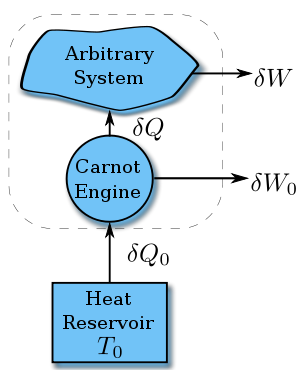# Clausius theorem

﻿
Clausius theorem

The Clausius theorem (1855) states that in a cyclic process$\oint \frac{\delta Q}{T} \leq 0.$

The equality holds in the reversible case and the '<' is in the irreversible case. The reversible case is used to introduce the state function entropy. This is because in cyclic process the variation of a state function is zero.

## ProofProving Clausius Inequality

Suppose a system absorbs heat δQ at temperature T. Since the value of$\frac{\delta Q}{T}$ does not depend on the details of how the heat is transferred, we can assume it is from a Carnot engine, which in turn absorbs heat δQ0 from a heat reservoir with constant temperature T0.

According to the nature of Carnot cycle,$\frac{\delta Q}{T}=\frac{\delta Q_0}{T_0}$$\Rightarrow\delta Q_0=T_0\frac{\delta Q}{T}$

Therefore in one cycle, the total heat absorbed from the reservoir is$Q_0=T_0\oint\frac{\delta Q}{T}$

Since after a cycle, the system and the Carnot engine as a whole return to its initial status, the difference of the internal energy is zero. Thus according to First Law of Thermodynamics,

Q0 = ΔU + W + W0 = W + W0 = Wtotal

According to the Kelvin statement of Second Law of thermodynamics, we cannot drain heat from one reservoir and convert them entirely into work without making any other changes, so$W_\text{total}\leq 0$

Combine all the above and we get Clausius inequality$\oint\frac{\delta Q}{T}\leq 0$

If the system is reversible, then reverse its path and do the experiment again we can get$-\oint\frac{\delta Q}{T}\leq 0$

Thus$\oint\frac{\delta Q}{T}=0$

Wikimedia Foundation. 2010.

### Look at other dictionaries:

• Clausius–Duhem inequality — Continuum mechanics …   Wikipedia

• Clausius , Rudolf Julius Emmanuel — (1822–1888) German physicist Clausius, who was born in Köslin (now Koszalin in Poland), studied at the University of Berlin and obtained his doctorate from Halle in 1848. He was professor of physics at the Royal Artillery and Engineering School,… …   Scientists

• Virial theorem — In mechanics, the virial theorem provides a general equation relating the average over time of the total kinetic energy, , of a stable system consisting of N particles, bound by potential forces, with that of the total potential energy, , where… …   Wikipedia

• virial theorem — noun A theorem in mechanics showing the relationship between the kinetic energy of a system to the virial of Clausius …   Wiktionary

• Entropy — This article is about entropy in thermodynamics. For entropy in information theory, see Entropy (information theory). For a comparison of entropy in information theory with entropy in thermodynamics, see Entropy in thermodynamics and information… …   Wikipedia

• Entropy (classical thermodynamics) — In thermodynamics, entropy is a measure of how close a thermodynamic system is to equilibrium. A thermodynamic system is any physical object or region of space that can be described by its thermodynamic quantities such as temperature, pressure,… …   Wikipedia

• Thermodynamics — Annotated color version of the original 1824 Carnot heat engine showing the hot body (boiler), working body (system, steam), and cold body (water), the letters labeled according to the stopping points in Carnot cycle …   Wikipedia

• Список научных публикаций Альберта Эйнштейна — Альберт Эйнштейн (1879 1955) был известным специалистом по теоретической физике, который наиболее известен как разработчик общей и специальной теорий относительности. Он также внёс большой вклад в развитие статистической механики, особенно… …   Википедия

• Thermodynamic system — A thermodynamic system is a precisely defined macroscopic region of the universe, often called a physical system, that is studied using the principles of thermodynamics. All space in the universe outside the thermodynamic system is known as the… …   Wikipedia

• Non-equilibrium thermodynamics — Thermodynamics …   Wikipedia